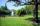# 9th grade (14y) + Heron's formula - math problems

1. Triangle ABCCalculate the sides of triangle ABC with area 1404 cm2 and if a: b: c = 12:7:18
2. DescribedCalculate perimeter of the circle described by a triangle with sides 478, 255, 352.
3. TrapezoidCalculate area of trapezoid ABCD with sides |AD|= 68 cm, |DC|=35 cm, |CB|=12 cm, |AB|=35 cm..
4. Triangle SSSCalculate perimeter and area of ​​a triangle ABC, if a=42, b=57 and c=42.
5. PrismCalculate the volume of the rhombic prism. Base of prism is rhombus whose one diagonal is 47 cm and the edge of the base is 28 cm. The edge length of the base of the prism and height is 3:5.
6. Cardboard boxWe want to make a cardboard box shaped quadrangular prism with rhombic base. Rhombus has a side of 5 cm and 8 cm one diagonal long. The height of the box to be 12 cm. The box will be open at the top. How many square centimeters cardboard we need, if we cal
7. Heron backlawCalculate missing side in a triangle with sides 17 and 34 and area 275.
8. ParkIn the newly built park will be permanently placed a rotating sprayer irrigation of lawns. Determine the largest radius of the circle which can irrigate by sprayer P so not to spray park visitors on line AB. Distance AB = 55 m, AP = 36 m and BP = 28 m.
9. Circles 2Calculate the area bounded by the circumscribed and inscribed circle in triangle with sides 12 cm, 14 cm, 18 cm.
10. TriangleCalculate heights of the triangle ABC if sides of the triangle are a=75, b=84 and c=33.
11. DiamondRhombus has side 17 cm and and one of diagonal 22 cm long. Calculate its area.
12. SailsWe known heights 220, 165 and 132 of sail. It has triangular shape. What is the surface of the sail?
13. TriangleThe triangle has known all three sides: a=5.5 m, b=5.3 m, c= 7.8 m. Calculate area of ​this triangle.
14. TriangleDetermine whether a triangle can be formed with the given side lengths. If so, use Heron's formula to find the area of the triangle. a = 158 b = 185 c = 201
15. The farmerThe farmer would like to first seed his small field. The required amount depends on the seed area. Field has a triangular shape. The farmer had fenced field, so he knows the lengths of the sides: 119, 111 and 90 meters. Find a suitable way to determine th
16. Sides ratioCalculate the circumference of a triangle with area 84 cm2 if a:b:c = 10:17:21
17. Sss triangleCalculate the area and heights in the triangle ABC by sides a = 8cm, b = 11cm, c = 12cm
18. One trapeziumOne trapezium has AB=24M, BC=36M, CD=80M, DA=80M long sides. Find the area.

Do you have an interesting mathematical word problem that you can't solve it? Enter it, and we can try to solve it.

To this e-mail address, we will reply solution; solved examples are also published here. Please enter the e-mail correctly and check whether you don't have a full mailbox.

Please do not submit problems from current active competitions such as Mathematical Olympiad, correspondence seminars etc...